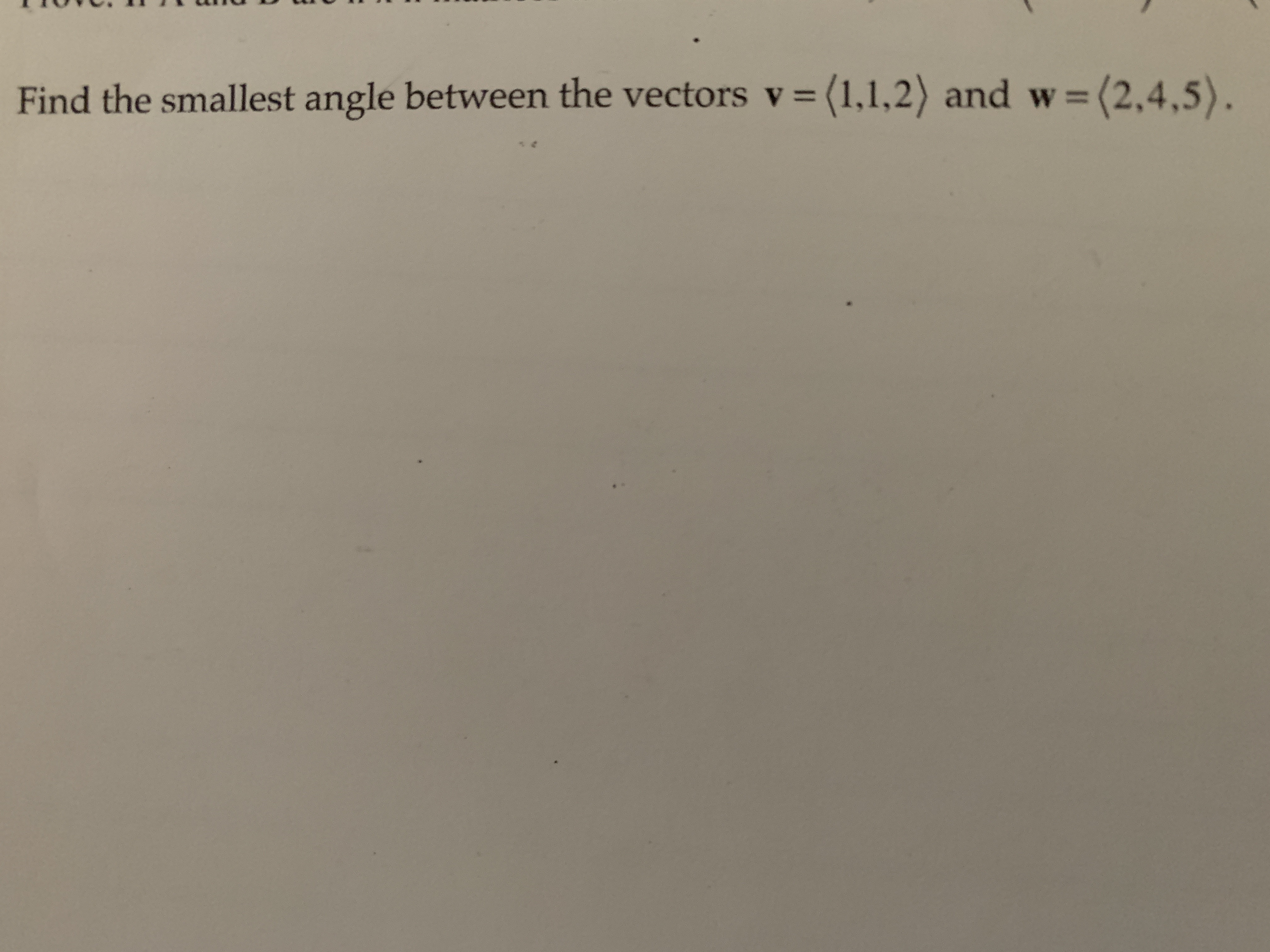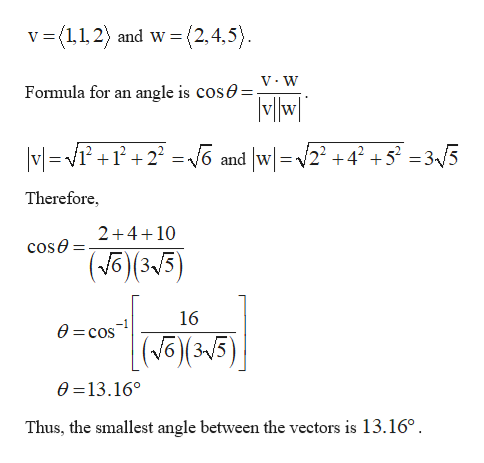Find the smallest angle between the vectors v = (1,1,2) and w= (2,4,5).

Questionhelp_outlineImage TranscriptioncloseFind the smallest angle between the vectors v = (1,1,2) and w= (2,4,5). fullscreen

The given vectors ...help_outlineImage Transcriptionclose(2,4,5) v (1,1,2 and w angle is COSA=V:W MWl Formula for an VG and w2 +42 +5? = 3«/5 MV2 Therefore 2+410 cose (315 16 0 cos |(J (315) 63V 0 13.16° Thus, the smallest angle between the vectors is 13.16° fullscreen

Want to see the full answer?

See Solution

Want to see this answer and more?

Our solutions are written by experts, many with advanced degrees, and available 24/7

See Solution
Tagged in

Math